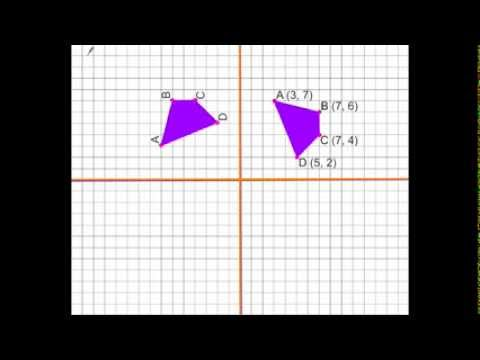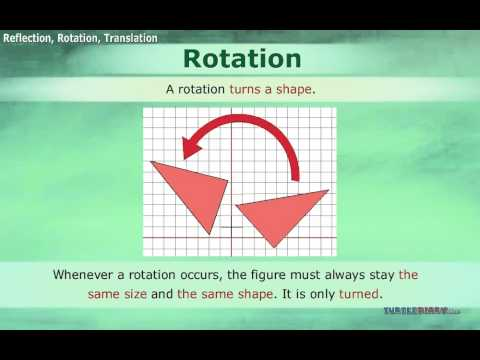## Rotation of Geometrical Figures

Subject: Compulsory Maths

#### Overview

When each vertex of a geometrical figure is rotated through a certain angle in a certain direction about a given centre of rotation the figure is said to be rotated through the same centre of rotation. When the coordinates of the image of a point is rotated through 90 and 180 in anticlockwise and clockwise directions about the centre of rotation at origin.

#### Rotation of geometrical figures

When each vertex of a geometrical figure is rotated through a certain angle in a certain direction about a given centre of rotation the figure is said to be rotated through the same centre of rotation.

To rotate a geometrical figure, following three conditions are required

1. Centre of Rotation
2. Angle of Rotation
3. Direction of Rotation

A figure can be rotated in two directions

1. Anti-clockwise direction
2. Clockwise direction

Rotation through 90° in anticlockwise direction

1. Join each vertex of the figure to the centre of rotation with dotted lines.
2. On each dotted lines draw 90° at O with the help of a protractor in an anticlockwise direction.
3. With the help of compass, cut off OA' = OA, OB' = OB and OC' = OC.
4. Join A', C', and C'.

Therefore, $\triangle$A'B'C' is the image of $\triangle$ABC formed due to the rotation through 90° in the anticlockwise direction about 0.Rotation through 90° in clockwise direction

Those similar steps are followed which are mentioned above. While making the angle of 90° you should draw it in the clockwise direction. In the diagram, $\triangle$A'B'C' is the image of $\triangle$ABC formed due to the rotation through 90° in a clockwise direction about O. In a similar way, we can rotate geometrical figure through 180° in an anti-clockwise or clockwise direction about the given centre of rotation.

Rotation of Geometrical figures using coordinates

When the coordinates of the image of a point are rotated through 90° and 180° in anticlockwise and clockwise directions about the centre of rotation at an origin.

Rotation through 90° in clockwise about origin

When a point is rotated through 90° in an anti-clockwise direction about the origin as the centre of rotation, the x and y coordinates are exchanged by making the sign of y coordinates just opposite.

i.e P(x,y)→P'(-y,x)

Rotation through 90° in clockwise about origin

When a point is rotated through 90° in the clockwise direction about the origin as the centre of rotation, the x and y coordinates are exchanged by making the sign of x coordinates just opposite.

i.e P(x,y)→P'(y,-x)

Rotation through 180° in anticlockwise and clockwise about origin

When a point is rotated through 180° about origin the coordinates of the image are the same in both directions.When a point is rotated through 180° in anticlockwise or in a clockwise direction about the origin as the centre of rotation the x and y coordinates of the image remain same just by changing their signs.

i.e P(x,y)→P'(-x,-y)

Function machine and relation between the variables x and y

Observe the diagram of a function machine. When different numbers are inserted into the machine, it does the certain process to bring out results under the certainly given rule. Here, the inserted numbers are called inputs and the result coming out from the machine are called outputs.

##### Things to remember
• Rotation through 90 in anticlockwise direction

1. Join each vertex of the figure to the centre of rotation with dotted lines.
2. On each dotted lines draw 90 at O with the help of a protractor in an anticlockwise direction.
3. With the help of compass, cut off OA' = OA, OB' = OB and OC' = OC.
4. Join A', C', and C'.
• It includes every relationship which established among the people.
• There can be more than one community in a society. Community smaller than society.
• It is a network of social relationships which cannot see or touched.
• common interests and common objectives are not necessary for society.
##### Videos for Rotation of Geometrical Figures##### Geometric Transformations - Rotations##### Translations Reflections and Rotations

Solution:

A(2,1)$\rightarrow$A’(-1,2)

B(4,3)$\rightarrow$B’(-3,4)

C(1,4)$\rightarrow$C’(-4,1)

So, the coordinates of the vertices of image $\triangle$A’B’C’ are A(-1,2), B(-3,4) and C(-4,1).

Solution:

Here, when input is 1, output = 4$\rightarrow$1 + 3 $\rightarrow$ input + 3

When input is 2, output = 5$\rightarrow$2 + 3 $\rightarrow$input +3

When input is 3, output = 6$\rightarrow$3 + 3$\rightarrow$input+3

Similarly, when input is x, output = x+3

$\therefore$ The required relation is y = x + 3.

Now, when x = 4, y = 4+3 = 7

When x= 5, y = 5+3 = 8

When x = 6, y = 6+3 = 9

When x = 7, y = 7+3 = 10.

Solution:

Here, the relation is y = 2x – 1

When x = 1, y = 2×1-1 =`1; when x = 2, y = 2×2-1 = 3

When x = 3, y = 2×3-1 = 5; when x = 4, y = 2×4-1 = 7

 Input (x) 1 2 3 4 Output (y) 1 3 5 7